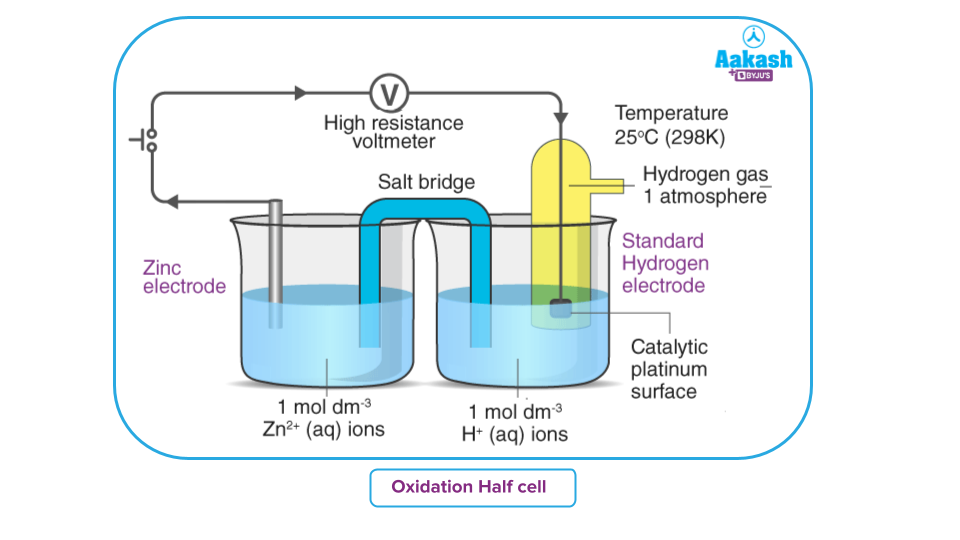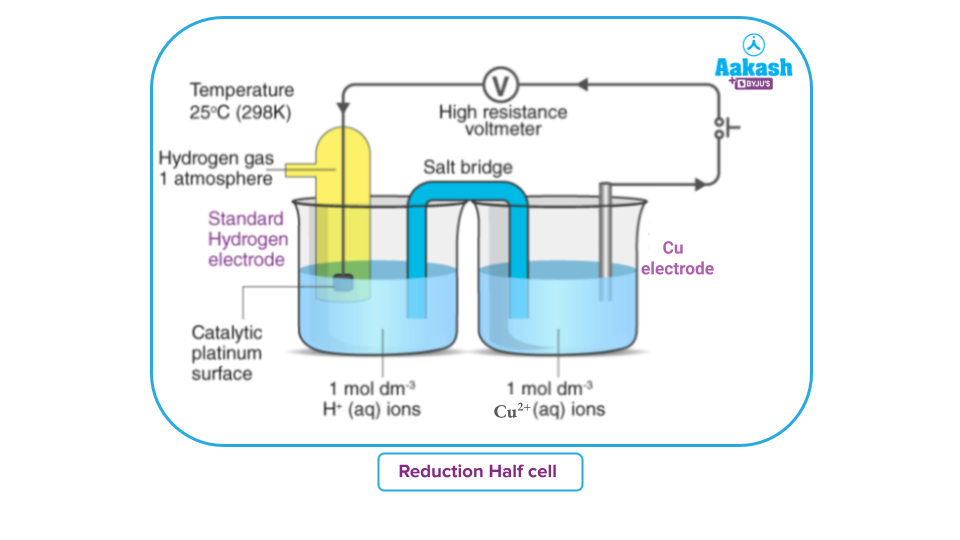• Call Now

1800-102-2727•

# Standard Electrode Potential: Definition of Standard Electrode Potential, Calculation of Standard Electrode Potential, Practice Problems, FAQs

Have you ever been to any grocery store to buy something?

Imagine you went to a grocery store to buy 2kg of sugar. The shopkeeper took the weight and placed it on the weighing balance. On the other side of the balance, he started putting sugar units at both ends to reach equilibrium.

You gave him the required amount of money and came back home.

How do you know whether it is exactly 2kg or not?

Well, you can trust the shopkeeper as they only use standardised weights, which are verified.

By standardising a particular weight we can calculate the weight of an unknown substance.

Similarly in electrochemistry, we can measure standard electrode potential at a standard condition.

Let’s understand how can we calculate standard electrode potential and its application.Table of Content

• Definition of Standard Electrode Potential
• The Importance of a Standard Electrode Potential
• Redox Reactions' Spontaneity
• Calculation of Standard Electrode Potential of Zn/Zn2+ Electrode
• Calculation of Standard Electrode Potential of Cu/Cu2+ Electrode
• Practice Problems

## Definition of Standard Electrode Potential

An electrochemical cell can be considered as made of two half cells. In one half cell oxidation takes place and in the other reduction takes place. By convention, we show oxidation reaction and the anode on the left side and the reduction half-cell and cathode on the right side.

The electrode potential is referred to as the standard electrode potential when the concentrations of all the species in an electrode half-cell are under standard conditions.

An electrochemical cell operates at the standard electrode potential when the following conditions are present:

• temperature = 298K pressure = 1atm concentration = 1M

Standard electrode potential of a cell is generally denoted by Ecell

## The Importance of a Standard Electrode Potential

• The foundation of any electrochemical cell is the redox process, which consists of two parts.
• The oxidation half-reaction, which involves the loss of electrons, occurs at the anode.
• An electron gain results from a reduction process that takes place at the cathode. As a result, electrons move from the anode to the cathode.
• The difference in the unique potentials of each electrode is what determines the electric potential between the anode and the cathode.
• It is measured using a typical hydrogen electrode as a reference electrode. Standard Hydrogen Electrode has an electrode potential of 0 volts.
• The cell potential of the ensuing galvanic cell, created when an electrode is connected to the Standard Hydrogen Electrode, can be utilised to determine the electrode's standard electrode potential.
• An electrode's oxidation potential is the opposite of its reduction potential. As a result, an electrode's standard electrode potential can be described by the standard reduction potential of the electrode.
• Oxidizing ability increases with increasing standard reduction potentials, while the reducing nature decreases with increasing standard reduction potentials.
• Ca2+ has a typical electrode potential of -2.87 V, while F2 has a potential of +2.87 V. As a result, Ca is a great reducing agent and F2 is a great oxidising agent.

## Redox Reactions' Spontaneity

If a redox reaction occurs spontaneously, the ΔG0 (Gibbs free energy) must be negative. The following equation describes it:

Where

• n = the total amount of electron moles needed to produce one mole of product.
• F = Faraday's constant (96485 C.mol-1).

The E0cell may be calculated using the following equation:

${{E}^{0}}_{cell}={{E}^{0}}_{cathode}-{{E}^{0}}_{anode}$

As a result, E0cell may be calculated by subtracting the anode's standard electrode potential from the cathode's. The E0cell must be +ve for a redox reaction to be spontaneous (since both n and F have positive values, and the ΔGvalue must be -ve).

This suggests that in a natural process,

The conventional electrode potentials for the cathode and anode help predict the spontaneity of the cell reaction as a result. It is worth noting that the cells ΔG0 is -ve in galvanic cells and +ve in electrolytic cells.

## Calculation of Standard Electrode Potential of $\frac{Zn}{Z{n}^{2+}}$ Electrode

The difference in potential between the electrode and the electrolyte is known as the potential of the electrode and is measured by coupling the electrode with a reference standard electrode which is mostly a Standard Hydrogen electrode.

A standard zinc electrode is the zinc metal rod dipped in a 1 M ZnSO4 solution..

The standard half-cell potential of an electrode is measured by coupling the required electrode with a standard hydrogen electrode (SHE). SHE is a gas electrode. SHE consists of a thin platinum foil coated with platinum black serving the electrode. the platinum electrode is dipped in a solution containing 1.00M hydrogen ions through which hydrogen gas at one-atmosphere pressure is continuously bubbled.

SHE is assumed to have a standard potential of zero volts. The potential measured will be then the potential of the electrode.

Depending upon the redox reaction that the electrode can undergo, The electrode can behave as a cathode or anode.

Let us consider a zinc electrode coupled with SHE to form a cell.Zinc is more electropositive than hydrogen. Hence the hydrogen ion undergoes a reduction reaction with a zinc electrode and acts as a cathode, while zinc undergoes oxidation and acts as an anode.

(Reduction Occurs)

Oxidation:

The above cell's EMF is measured as 0.76 volt.

Consequently, the $\frac{Zn}{Z{n}^{2+}}$electrode's standard electrode potential is 0.76 volt. In addition, the flow of the current indicates that oxidation takes place at the zinc electrode. Therefore, the $\frac{Zn}{Z{n}^{2+}}$ electrode's standard oxidation potential is 0.76 volt.

## Calculation of Standard Electrode Potential of $\frac{Cu}{C{u}^{2+}}$ Electrode

A standard hydrogen electrode and a copper electrode dipped in a 1 M CuSO4 solution are used to set up a cels.Hydrogen is more electropositive than Copper. Hence SHE will undergo oxidation and function as an anode:

${H}_{2}\to 2{H}^{+}+2{e}^{-}$ (Oxidation occurs)

Copper electrode acts as a cathode and copper ions from the electrolyte get reduced.

Reduction:

EMF of the cell comes out to be 0.34 volt. Hence, the standard electrode potential of $\frac{Cu}{C{u}^{2+}}$ electrode is 0.34 volt.

Additionally, the current's direction of flow suggests that reduction occurs at the copper electrode. Hence, 0.34 volt is the standard reduction potential of $\frac{Cu}{C{u}^{2+}}$the electrode.

Point to be remembered:

Since the reduction half-reaction is just the reverse of the oxidation half-reaction, therefore, the reduction potential is obtained from the oxidation potential by simply changing the sign, i.e., for any electrode.

Reduction potential= - Oxidation potential

It is common practice to refer to reduction potential always and change to oxidation potential if needed using the above relation.

Thus, for the zinc electrode, Standard Reduction Potential = - 0.76 V

A positive value of the standard electrode potential for the copper electrode indicates that Cu2+ ions gets reduced more easily than H+ ions under standard conditions. In other words, Cu can’t be oxidised to Cu2+ ions in the presence of H+ ions. Hence, copper does not dissolve in HCl. it may be noted that in the case of nitric acid, copper is oxidised by nitrate ions and not by H+ ions.

## Practice Problems

Q1 What will be the value of a $\Delta {\mathbit{G}}^{0}$, if the reaction is spontaneous?

A. Positive
B. Negative
C. Infinite
D. Can’t be predicted

Solution: A substance's available energy that can be applied in a chemical reaction or transformation is known as the Gibbs free energy. Substances frequently change into other substances with lower Gibbs free energies. Whether a chemical reaction will take place spontaneously is predicted by the change in Gibbs free energy. A negative value of Gibbs free energy indicates that the reaction is spontaneous.

Q2 Electrode potential determines the _______

A. Kinetic of a cell reaction
B. Thermodynamics of a cell reaction
C. Tendency of the electrode to lose or gain an electron
D. None of the above

Solution: Electrode potential is defined as the electromotive force (EMF) developed in a galvanic cell made with the electrode against a standard reference electrode. It is denoted by E and the unit of electrode potential is volt. The tendency for an electrode to gain or lose electrons when in contact with its own ionic solution.

Q3 Stronger the oxidizing agent, the greater will be the:

A. Oxidation potential
B. Reduction potential
C. Both A & B
D. None of the above

Solution: The property of an oxidizing agent is to oxidize other but itself it should get reduced. The tendency of an electrode to gain electrons or to get reduced is known as its reduction potential. Hence, the greater the value of reduction potential, the stronger the oxidizing agent.

Q4. Which of the following is not a factor that can affect the value of electrode potential?

A. Nature of metal or electrode.
B. The concentration of metal ions in solution.
C. Temperature.
D. Size of the vessel

Solution: Electrode potential is generally affected by the following factors

• Nature of metal or electrode.
• The concentration of metal ions in solution.
• Temperature.

Q1. What is the meaning of electrode potential?
The potential of an electrode is its ability to either get oxidised or reduced while in contact with its salt solution. It is measured as the potential of a cell, having the electrode in question with a standard hydrogen electrode. The electrode has a positive reduction potential when it acts as an anode and negative reduction potential (or positive oxidation potential) when it acts as a cathode.

Q2. What is the distinction between standard electrode potential and standard cell potential?
The standard reduction potential is found in a class known as normal cell potential or standard electrode potential. The inherent potential of cells is the potential difference between the cathode and anode. All standard potentials are measured at 298 K, 1 atm, and 1 M solutions.

Q3. What factors influence electrode potential?
The electrode potential is influenced by the concentration of metal ions in the electrode cell, pressure and temperature.

Q4. What improves the potential of cells?
By raising the concentration of one of the electrolyte solutions, you increase the number of cations and anions (depending on which electrolyte you raise), and hence the voltage potential of the cell.Talk to our expert
Resend OTP Timer =
By submitting up, I agree to receive all the Whatsapp communication on my registered number and Aakash terms and conditions and privacy policy